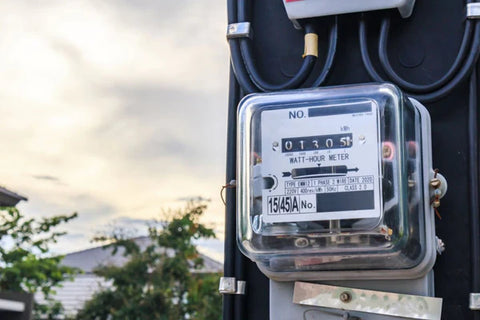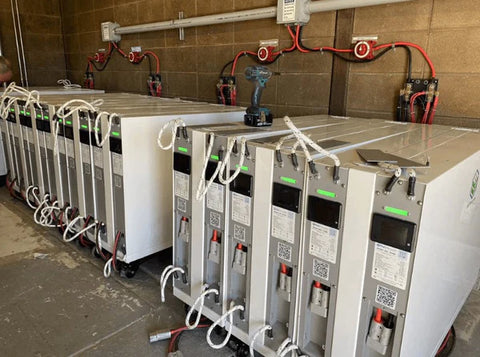Amps to Kilowatts (kW) Calculator - ShopSolar.com

# Amps to Kilowatts (kW) Calculator

As solar enthusiasts, we often find ourselves working with many different units of measurement. It’s crucial, of course, since we need the electrical current to flow correctly and power everything we need it to.

One of the units we’re going to work with is amps, but what if we need to convert it to kilowatts?  How do we do this and why is it important?

While most electrical equipment has a label that bares all the specs and units we need to know, this isn’t always the case. At times like these, you’ll need to do a conversion since different units measure different aspects.

You’ll most likely need to use conversions when you’re working with small solar panel kits and the like. Below we’ll be looking at how to go about converting Amps to Kilowatts with the amps to kilowatts calculator.

kW
W
mW

## Why We Use Conversions

We typically convert amps to watts when doing calculations since it’s easier to work with numbers and amounts that use the same kind of units. This also prevents miscalculations because of the differences between different units of measurement.

Amps and watts are often used when calculating how much power is being consumed by your household appliances and lighting fixtures.

Although watts and amps are units for measuring power, exactly what they measure is significantly different. Watts measure the total amount of energy in a system, whereas Amps simply measure the amount of current being drawn.

There are a few units of measurement that are going to appear throughout the article, as it’s only natural since we’re discussing how to measure certain units and how to convert them.

### kW (Kilowatts)

Kilowatts, also known as working power, are the actual amount of energy an appliance needs to operate. Using values that have been converted into kilowatts is easier to work with because of how they measure energy.

### Amps (Amperes)

Figuring out your circuit’s current in amps tells you how much electricity is flowing through it. It allows you to monitor its performance and make sure that it’s operating as it needs to.

## How to Convert Amps to Kilowatts Using the CalculatorConverting amps to kilowatts can be done using a formula derived from Watt’s Law formula. The original formula looks like this:

I = P ÷ V

In this formula, P represents the power in Watts, I is the current in Amps, and V is the voltage in Volts. To convert amps to kilowatts, the modified formula shows us that the power (kilowatts) is equal to the current (amps) multiplied by the voltage (volts), which is then divided by 1,000.

So, the formula is written in the following way:

Kilowatts = (Amps ✕ Volts) ÷ 1,000

### Amps to Kilowatts (kW) Calculator Example

We can use this method to figure out how many watts a battery bank like this 48v LiFePo4 battery is able to generate. The maximum discharging current this kind of battery is able to generate is 150A, and it has a voltage range between 43V and 58V. For the next example, we’ll be using the maximum voltage, 58V.

Kilowatts = (150A ✕ 58V) ÷ 1,000

Kilowatts = 8,700 ÷ 1,000

Kilowatts = 8.7kW

Using the formula allows you to get an accurate idea of what the battery is capable of at maximum performance, although the voltage might change according to how you’re using it.

Not to mention, in the unlikely event that the label on the battery itself doesn’t show the number of Kilowatts it generates, using the formula gets you as close to the real number as possible.

### Converting Amps to Kilowatt-Hours

Before diving in, we should first convert amps into amp-hours, which is easy enough since we’re just multiplying the amps we’re using by one hour. The formula looks like this:

Amp-hours (Ah) = Amps (A) ✕ Hours (H)

For example, the battery in question has a maximum continuous discharge of 150 Amps, which we then multiply by an hour. Even if you’ve just used the battery for a few minutes, you’ll still have to multiply by the hour. Let’s say you’ve used the battery for 45 minutes, the formula would look like this:

Ah = 150A ✕ 0.75 Hours

Ah = 112.5 Amp-hours

With our nifty battery amp-hour calculator out of the way, we can now move on to the main topic at hand - kilowatt-hours. Generally, battery sizes are listed in amp-hours, which helps when comparing batteries of the same voltages.

Although, the confusion only sets in once you start comparing the amp-hours of batteries with different voltages. This is usually because the total amount of energy that a battery can generate depends on its Amp-hours and voltage.To avoid confusion, we need to know how to convert amp-hours to watt-hours. One we know this, we can convert amp-hours to kilowatt-hours, which can be done using a formula and the Amp-hours we got from the battery – we’ll just round up the result.

Kilowatt-hours (kWh) = Amp-hours (Ah) ✕ Volts (V) ÷ 1,000

kWh = 113 Ah ✕ 48 V ÷ 1000

kWh =  5.4 kWh

The example in this formula shows us that the battery size is around 5 Kilowatt-hours, which is enough to power more than a few things in your home like a fridge, TV, and even a few lights – it’s also enough to last for 10 hours.

### Converting Amps to Milli-Amps

Milliamps (mA) are units of measurement for current flowing through an electrical conductor as well as the rate at which the electrons are flowing. A milliamp is one-thousandth of an Ampere, which is usually displayed as 0.001 Amps.We can often see the unit being used for batteries, especially phone batteries. The average battery capacity that can be found in our phones is around 4001mAh. Converting amps to milliamps is easy since you can either divide or multiply by 1,000 to determine both Amps and Milliamps.

You can find all of the we have created in order to help you plan, size and install your own solar power system here.

## Join the Solar Lab!